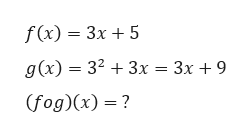f(x)=3x+5 and g(x)=32+3x.After simplifying,(f∘g)(x)=

Question

f(x)=3x+5 and g(x)=32+3x.
After simplifying,
(f∘g)(x)=

Step 1

Given,

...help_outlineImage Transcriptionclosef(x) 3x5 g(x) 323x = 3x + 9 (fog)(x)? fullscreen

Want to see the full answer?

See Solution

Want to see this answer and more?

Our solutions are written by experts, many with advanced degrees, and available 24/7

See Solution
Tagged in

Other